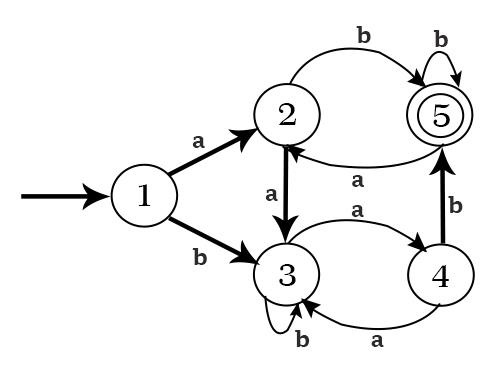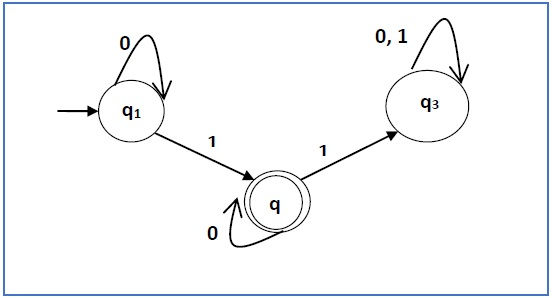# Arden's Theorem

In order to find out a regular expression of a Finite Automaton, we use Arden’s Theorem along with the properties of regular expressions.

Statement

Let P and Q be two regular expressions.

If P does not contain null string, then R = Q + RP has a unique solution that is R = QP*

Proof

R = Q + (Q + RP)P [After putting the value R = Q + RP]

= Q + QP + RPP

When we put the value of R recursively again and again, we get the following equation −

R = Q + QP + QP2 + QP3…..

R = Q (ε + P + P2 + P3 + …. )

R = QP* [As P* represents (ε + P + P2 + P3 + ….) ]

Hence, proved.

## Assumptions for Applying Arden’s Theorem

• The transition diagram must not have NULL transitions
• It must have only one initial state

### Method

Step 1 − Create equations as the following form for all the states of the DFA having n states with initial state q1.

q1 = q1R11 + q2R21 + … + qnRn1 + ε

q2 = q1R12 + q2R22 + … + qnRn2

…………………………

…………………………

…………………………

…………………………

qn = q1R1n + q2R2n + … + qnRnn

Rij represents the set of labels of edges from qi to qj, if no such edge exists, then Rij = ∅

Step 2 − Solve these equations to get the equation for the final state in terms of Rij

Problem

Construct a regular expression corresponding to the automata given below −Solution

Here the initial state and final state is q1.

The equations for the three states q1, q2, and q3 are as follows −

q1 = q1a + q3a + ε (ε move is because q1 is the initial state0

q2 = q1b + q2b + q3b

q3 = q2a

Now, we will solve these three equations −

q2 = q1b + q2b + q3b

= q1b + q2b + (q2a)b (Substituting value of q3)

= q1b + q2(b + ab)

= q1b (b + ab)* (Applying Arden’s Theorem)

q1 = q1a + q3a + ε

= q1a + q2aa + ε (Substituting value of q3)

= q1a + q1b(b + ab*)aa + ε (Substituting value of q2)

= q1(a + b(b + ab)*aa) + ε

= ε (a+ b(b + ab)*aa)*

= (a + b(b + ab)*aa)*

Hence, the regular expression is (a + b(b + ab)*aa)*.

Problem

Construct a regular expression corresponding to the automata given below −Solution

Here the initial state is q1 and the final state is q2

Now we write down the equations −

q1 = q10 + ε

q2 = q11 + q20

q3 = q21 + q30 + q31

Now, we will solve these three equations −

q1 = ε0* [As, εR = R]

So, q1 = 0*

q2 = 0*1 + q20

So, q2 = 0*1(0)* [By Arden’s theorem]

Hence, the regular expression is 0*10*.